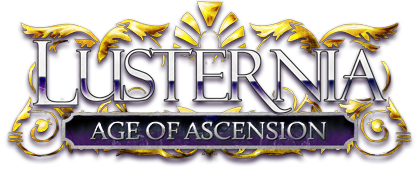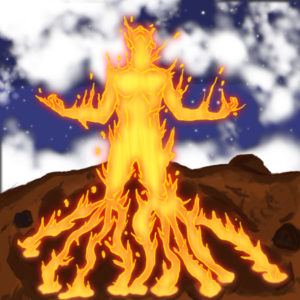### Article One

This is where we describe the awesomeness of the article. This is where we describe the awesomeness of the article. This is where we describe the awesomeness of the article. This is where we describe the awesomeness of the article.### Article Two

This is where we describe the awesomeness of the article. This is where we describe the awesomeness of the article. This is where we describe the awesomeness of the article. This is where we describe the awesomeness of the article.

## Calendar

• July 9: Wild Nodes!
• July 23: Wild Nodes!